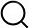# Also in the Article

Homophilic connectivity

Constructing graphs from genetic encodings
Sci Rep, Jun 24, 2021;

Procedure

The structure of the investigated wiring rules lends itself to a model of homiphilic connectivity, a popular consideration in social networks. Specifically, we aim to connect nodes with similar identities, which can be achieved by placing the same identities on either side of a rule: $(010XX)O(010XX)$. While previously we considered rules where nodes with similar identities share connectivity patterns, we now aim to connect nodes with similar identities to each other. In the language of the wiring rules, until now we focused on rules of the sort $(010XX)O(0X01X)$, where the two sets to be connected may or may not share nodes, while with homiphily rules we consider rules of the form $(010XX)O(010XX)$, where we connect the same two sets of nodes.

Although many possible approaches exist for modeling homiphily, we consider a simple scaling, where we connect all sets of nodes with at least $o=b-x$ overlap in their identities. For instance, $(010XX)O(010XX)$ would represent a rule from $o=3$, where nodes need at least 3 shared bits to connect. The extremes of this model lie at $o=0$, where no bits need to be shared, thus we have a fully connected graph, and $o=b$, where all bits need by shared, thus we have a network with only self loops. The interesting regime lies in $0, where the networks are expected to densify and have lower path length as o decreases. Simulations confirm these predictions (Fig. S5), and a stereotyped scaling emerges in density, clustering and path length over a number of system sizes.

Q&A